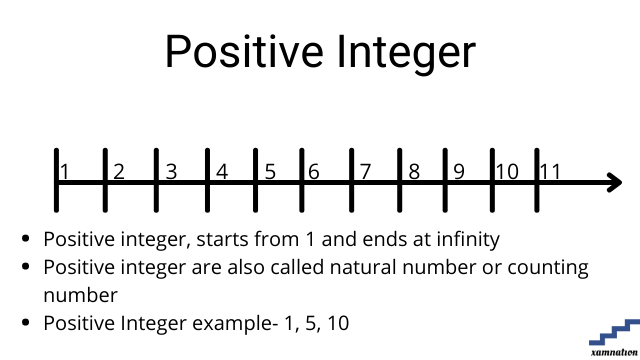# Maths for Grade 7 – Number System | what is Rational Numbers | what are Positive and Negative Integer | what is non-negative Integer

Number system tutorial

Number system is system of representing numbers in mathematics. Students deal with various types of numbers for solving mathematics formulas and calculation, data processing and handling complex topics like algebra and geometry.

In this article, we have shared different types of numbers that are used in mathematics like Rational Numbers, Positive, Negative and non-negative Integer. This article can be used by all grades students, as the definition of these different types of numbers is same for all grades.

## What is Rational Number?

### Definition of whole number

• Any number that can be expressed as the quotient or fraction p/q, where p and q are integers.
• It can be positive , negative or 0
• It cannot be integer or decimal number### Examples of rational number

• 4/5 is a rational number where it is represented as numerator (4) and denominator (5)
• 5/4, 1/3, -2/5 are rational numbers
• -5, 1.6 are not whole numbers

Practice question on rational number

Can you find whether below mentioned numbers are rational or not?

• 10
• 5
• 10/13
• -1.1
• -2/3
• 0
• 305

Send your solution of finding rational numbers to info@xamnation.com , and know detailed answer of this problem.

## What is Positive Integer?

### Definition of Positive Integer

• Any number on the number line (1 and above) is called positive Integer.
• Positive Integer starts from 1 and end till infinity
• Zero and negative numbers are not positive integer### Examples of Integer

• 5, 10, 500, 3500 are positive Integer
• 0, -5, -20, -45 are not positive integer
• 6 , 1/3 are not positive Integer

Practice questions on positive Integer

Can you find whether below mentioned numbers is Integer or not?

• 10
• 5
• -15
• 10/13
• -1.1

Send your solution of finding positive Integer to info@xamnation.com , and know detailed answer of this problem.

## What is Negative Integer?

### Definition of Negative Integer

• Any number on the number line (-1 and below) is called negative Integer.
• Negative Integer starts from -1 and end till minus infinity
• Zero and positive numbers are not negative integer### Examples of negative Integer

• 0,5, 10, 500, 3500 are not negative Integer
• -5, -20, -45 are negative integer
• 6 , 1/3 are not negative Integer

Practice questions on negative Integer

Can you find whether below mentioned number is negative integer or not?

• 10
• 5
• -15
• 10/13
• -1.1

Send your solution of finding negative Integer to info@xamnation.com , and know detailed answer of this problem.

## What is Non Negative Integer?

### Definition of Positive Integer

• Any number on the number line (0 and above) is called non negative Integer.
• Non negative Integer starts from 0 and end till infinity
• Negative numbers are not positive integer### Examples of non-negative Integer

• 0, 5, 10, 500, 3500 are non-negative Integer
• -5, -20, -45 are not
• 6 , 1/3 are not

Practice questions on non-negative Integer

Can you find whether below mentioned numbers is non-negative Integer or not?

• 10
• 5
• -15
• 10/13
• -1.1

Send your solution of finding non-negative Integer to info@xamnation.com , and know detailed answer of this problem.

## Conclusion

In this article, we have seen how to find whether number is rational number, positive, negative and non-negative Integer. We also saw detailed examples and practical exercises for number system for grade 7 mathematics.

Are you struck in number system problem or any mathematics topics for grade 7 and above.

You can reach out to us for your math doubts and problem; our math tutors will help you in solving your doubts and take one-one math sessions online. Reach out to us, and our qualified math tutors for grade 7 and above will be glad to help you.